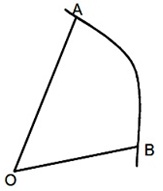#### Motion in more than one Dimension, Physics tutorial

Displacement, Velocity and Acceleration:

Let us suppose the motion of the particle in space.If particle is at position A at some instant of time t and at position B at another instant of time t + Dt. Position of the particle in the particular frame of reference is provided by the position vector drawn from origin of coordinate system in that frame to the position of particle. In the given diagram, let position vectors of A and B with respect to 0 be respectively. Displacement of particle in time interval is equivalent to in the direction AB. Therefore, average velocity of the particle during the time Δt is given by

Vav = Δr/ Δt

The direction is the same as that of since Δt is a scalar quantity.

Velocity at which particle would have travelled distance AB in uniform and rectilinear motion during the time interval Δt.

Uniform Circular motion:The angle Θ varies linearly with time so we can say where Θ = ωt where ω is constant. It is frequently referred to as angular velocity as it's the rate an angle changes with time. In present example it is just some constant with dimensions of inverse time.

So now we can explain the position of centipede in terms of it's x and y components as seen in the figure. A little trigonometry gives the x = R cos Θ and y = R sin Θ. So substituting Θ = ωt and expressing result in terms of vectors:

r = R(cos (ωt) i^ + sin(ωt)j^)........... eq.1

Good, now we have a well defined mathematical expression for the position of the centipede as a function of time. We can now differentiate it once to obtain the velocity

V = Rw(-sin(ωt) i^+ cos(ωt)j^)........... eq.2

So what does this say about the speed? We know how to take the magnitude of a vector right? Remember Pythagaros? (Did you know he worshiped beans?) So we have

|V| = Rw √sin2 (ωt) + cos2(ωt) = Rw........... eq.3

So v = ωR. So that tells us how to relate this mysterious ω to v. Now we can differentiate the velocity again getting

a = -Rω2 (cos(ωt)i^ +sin(ωt)j^)........... eq.4

But notice this is the same as a = -ω2r. That says that the acceleration points in opposite direction to radius vector r. The acceleration is directing center of the circle. Its magnitude is just α = Rω2. But from eq.3 this just says:

α = v2/R

This is known as the centripetal acceleration.

Relative motion:

Relative motion is the computation of the motion of an object with regard to some other moving object. Therefore, the motion is not computed through the reference to earth; however is the velocity of the object in reference to the other moving object as when it were in a static state.

For illustration: A ball thrown upward while in a moving object like a bus, would be traveling similar speed with respect to the bus and would fall again in relation to that speed. When the ball stopped in mid-air rather, the ball would hit the back of the bus prior to falling to the floor. In this illustration, the relative motion of the ball was illustrated in respect to the bus. When the driver turned left, the ball would as well be moving left even when it was stationary on the floor. When the ball was illustrated in relation to a distinct point, like a pedestrian on the side-walk and the bus was moving at 40 mph, it would be traveling faster than the pedestrian.

Two objects moving in same direction then relative motion is object with greater speed - object with lesser speed.

Relative speed: Speed of object A (greater speed) - speed of object B (lesser speed)

Tutorsglobe: A way to secure high grade in your curriculum (Online Tutoring)

Expand your confidence, grow study skills and improve your grades.

Since 2009, Tutorsglobe has proactively helped millions of students to get better grades in school, college or university and score well in competitive tests with live, one-on-one online tutoring.

Using an advanced developed tutoring system providing little or no wait time, the students are connected on-demand with a tutor at www.tutorsglobe.com. Students work one-on-one, in real-time with a tutor, communicating and studying using a virtual whiteboard technology.  Scientific and mathematical notation, symbols, geometric figures, graphing and freehand drawing can be rendered quickly and easily in the advanced whiteboard.

Free to know our price and packages for online physics tutoring. Chat with us or submit request at info@tutorsglobe.com